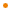# JavaFX: Bringing Rich Experiences To All the Screens Of Your Life

### Overview

A buffer that contains floating point data, intended for use as a parameter to effects such as DisplacementMap.

Profile: common conditional effect

### Variable Summary

accessnametypedescription
publicheightInteger1

The height of the map, in pixels.The height of the map, in pixels.

```       Min:    1
Max: 4096
Default:    1
Identity:  n/a
```

1
publicwidthInteger1

The width of the map, in pixels.The width of the map, in pixels.

```       Min:    1
Max: 4096
Default:    1
Identity:  n/a
```

1

### Function Summary

public setSample(x: Integer, y: Integer, band: Integer, s: Number) : Void

Sets the sample for a specific band at the given (x,y) location.Sets the sample for a specific band at the given (x,y) location.

Parameters
x
the x location
y
the y location
band
the band to set (must be 1, 2, 3, or 4)
s
the sample value to set

public setSamples(x: Integer, y: Integer, s0: Number) : Void

Sets the sample for the first band at the given (x,y) location.Sets the sample for the first band at the given (x,y) location.

Parameters
x
the x location
y
the y location
s0
the sample value to set for the first band

public setSamples(x: Integer, y: Integer, s0: Number, s1: Number) : Void

Sets the sample for the first two bands at the given (x,y) location.Sets the sample for the first two bands at the given (x,y) location.

Parameters
x
the x location
y
the y location
s0
the sample value to set for the first band
s1
the sample value to set for the second band

public setSamples(x: Integer, y: Integer, s0: Number, s1: Number, s2: Number) : Void

Sets the sample for the first three bands at the given (x,y) location.Sets the sample for the first three bands at the given (x,y) location.

Parameters
x
the x location
y
the y location
s0
the sample value to set for the first band
s1
the sample value to set for the second band
s2
the sample value to set for the third band

public setSamples(x: Integer, y: Integer, s0: Number, s1: Number, s2: Number, s3: Number) : Void

Sets the sample for each of the four bands at the given (x,y) location.Sets the sample for each of the four bands at the given (x,y) location.

Parameters
x
the x location
y
the y location
s0
the sample value to set for the first band
s1
the sample value to set for the second band
s2
the sample value to set for the third band
s3
the sample value to set for the fourth band